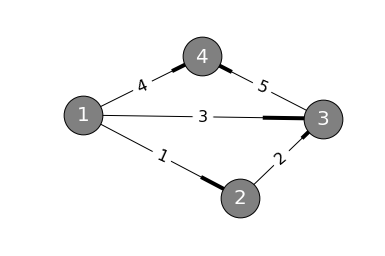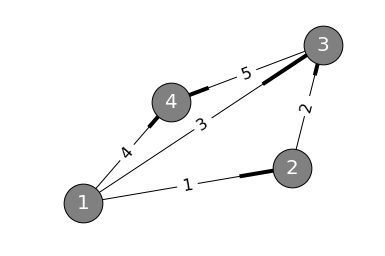# 第十二讲：图和网络

## 图和网络

import networkx as nx
import matplotlib.pyplot as plt
%matplotlib inline

dg = nx.DiGraph()
edge_labels = {(1, 2): 1, (1, 3): 3, (1, 4): 4, (2, 3): 2, (3, 4): 5}

pos = nx.spring_layout(dg)
nx.draw_networkx_edge_labels(dg,pos,edge_labels=edge_labels, font_size=16)
nx.draw_networkx_labels(dg, pos, font_size=20, font_color='w')
nx.draw(dg, pos, node_size=1500, node_color="gray")$\begin{array}{c | c c c c} & node_1 & node_2 & node_3 & node_4 \\ \hline edge_1 & -1 & 1 & 0 & 0 \\ edge_2 & 0 & -1 & 1 & 0 \\ edge_3 & -1 & 0 & 1 & 0 \\ edge_4 & -1 & 0 & 0 & 1 \\ edge_5 & 0 & 0 & -1 & 1 \\ \end{array}$

$$A^Ty=0=\begin{bmatrix}-1 & 0 & -1 & -1 & 0 \\1 & -1 & 0 & 0 & 0 \\0 & 1 & 1 & 0 & -1 \\0 & 0 & 0 & 1 & 1 \\ \end{bmatrix}\begin{bmatrix}y_1\\y_2\\y_3\\y_4\\y_5\end{bmatrix}=\begin{bmatrix}0\\0\\0\\0\end{bmatrix}$$，对于转置矩阵有$$dim N(A^T)=m-r=5-3=2$$

$电势差 \xrightarrow[欧姆定律]{矩阵C} 各边上的电流y_1, y_2, y_3, y_4, y_5 $$，而$$A^Ty=0$的另一个名字叫做“基尔霍夫电流定律”（Kirchoff's Law, 简称KCL）。

import networkx as nx
import matplotlib.pyplot as plt
%matplotlib inline

dg = nx.DiGraph()
edge_labels = {(1, 2): 1, (1, 3): 3, (1, 4): 4, (2, 3): 2, (3, 4): 5}

pos = nx.spring_layout(dg)
nx.draw_networkx_edge_labels(dg,pos,edge_labels=edge_labels, font_size=16)
nx.draw_networkx_labels(dg, pos, font_size=20, font_color='w')
nx.draw(dg, pos, node_size=1500, node_color="gray")$$A^Ty=0$$中的方程列出来： \left{ \begin{aligned} y_1 + y_3 + y_4 &= 0 \ y_1 - y_2 &= 0 \ y_2 + y_3 - y_5 &= 0 \ y_4 - y_5 &= 0 \ \end{aligned} \right.

• 现在假设$$y_1=1$$，也就是令$$1$$安培的电流在边$$1$$上流动；
• 由图看出$$y_2$$也应该为$$1$$
• 再令$$y_3=-1$$，也就是让$$1$$安培的电流流回节点$$1$$
• $$y_4=y_5=0$$

• $$y_1=y_2=0$$
• $$y_3=1$$
• 由图得$$y_5=1$$
• $$y_4=-1$$

$$N(A^T)$$的一组基为$$\begin{bmatrix}1\\1\\-1\\0\\0\end{bmatrix}\quad\begin{bmatrix}0\\0\\1\\-1\\1\end{bmatrix}$$

• $$y_3=0$$
• $$y_1=1$$
• 则由图得$$y_2=1, y_5=1, y_4=-1$$

$nodes-edges+loops=1$

• 将电势记为$$e$$，则在引入电势的第一步中，有$$e=Ax$$
• 电势差导致电流产生，$$y=Ce$$
• 电流满足基尔霍夫定律方程，$$A^Ty=0$$